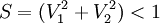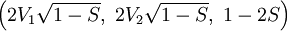Random vector on a sphere

The ability to generate a randomly orientated vector is very useful in Monte Carlo simulations of anisotropic models or molecular systems.

Marsaglia algorithm

This is the algorithm proposed by George Marsaglia 

• Independently generate V1 and V2, taken from a uniform distribution on (-1,1) such that$S=(V_1^2+V_2^2) < 1$
• The random vector is then (Eq. 4 in  ):$\left(2V_1 \sqrt{1-S},~ 2V_2 \sqrt{1-S},~ 1-2S\right)$

Fortran 90 implementation

This Fortran 90 implementation is adapted from Refs.  and . The function ran() calls a randon number generator:

!    The following is taken from Allen & Tildesley, p. 349
!    Generate a random vector towards a point in the unit sphere
!    Daniel Duque 2004

subroutine random_vector(vctr)

implicit none

real, dimension(3) :: vctr

real:: ran1,ran2,ransq,ranh
real:: ran

do
ran1=1.0-2.0*ran()
ran2=1.0-2.0*ran()
ransq=ran1**2+ran2**2
if(ransq.le.1.0) exit
enddo

ranh=2.0*sqrt(1.0-ransq)

vctr(1)=ran1*ranh
vctr(2)=ran2*ranh
vctr(3)=(1.0-2.0*ransq)

end subroutine random_vector